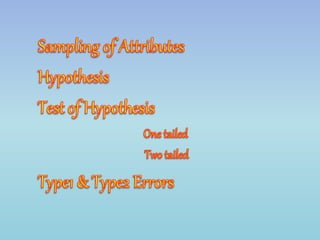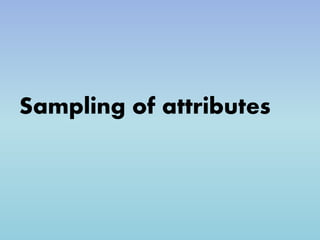Successfully reported this slideshow.

# Sampling of Attributes Hypothesis Test of Hypothesis One tailed Two tailed Type1 & Type2 Errors

12

Share×
1 of 18
1 of 18

# Sampling of Attributes Hypothesis Test of Hypothesis One tailed Two tailed Type1 & Type2 Errors

12

Share

HYPOTHESIS
A hypothesis is an idea or explanation that you then test through study and experimentation. Outside of science, theory or guess can also be called a hypothesis. A hypothesis is something more than a wild guess but less than a well-established theory.
.
.
.
Types
1)one-tailed
2)Two-tailed
.
.
One-tailed test alludes to the significance test in which the region of rejection appears on one end of the sampling distribution. It represents that the estimated test parameter is greater or less than the critical value. When the sample tested falls in the region of rejection, i.e. either left or right side, as the case may be, it leads to the acceptance of the alternative hypothesis rather than the null hypothesis.
..
.
.
examples...

HYPOTHESIS
A hypothesis is an idea or explanation that you then test through study and experimentation. Outside of science, theory or guess can also be called a hypothesis. A hypothesis is something more than a wild guess but less than a well-established theory.
.
.
.
Types
1)one-tailed
2)Two-tailed
.
.
One-tailed test alludes to the significance test in which the region of rejection appears on one end of the sampling distribution. It represents that the estimated test parameter is greater or less than the critical value. When the sample tested falls in the region of rejection, i.e. either left or right side, as the case may be, it leads to the acceptance of the alternative hypothesis rather than the null hypothesis.
..
.
.
examples...

## More Related Content

### Related Books

Free with a 14 day trial from Scribd

See all

### Related Audiobooks

Free with a 14 day trial from Scribd

See all

### Sampling of Attributes Hypothesis Test of Hypothesis One tailed Two tailed Type1 & Type2 Errors

1. 1. Sampling of attributes
2. 2. HYPOTHESIS Hypothesis is an idea or explanation that you then test through study and experimentation. Outside science, a theory or guess can also be called a hypothesis. A hypothesis is something more than a wild guess but less than a well-established theory.
3. 3. Testing of HYPOTHESIS It deals with the verification of validity of presumptions regarding the parameters of the population using samples drawn from population.
4. 4. Types 1)one tailed 2)Two tailed
5. 5. Definition of One-tailed Test One-tailed test alludes to the significance test in which the region of rejection appears on one end of the sampling distribution. It represents that the estimated test parameter is greater or less than the critical value. When the sample tested falls in the region of rejection, i.e. either left or right side, as the case may be, it leads to the acceptance of alternative hypothesis rather than the null hypothesis.
6. 6. • In this statistical hypothesis test, all the critical region, related to α, is placed in any one of the two tails. One-tailed test can be: • Left-tailed test: When the population parameter is believed to be lower than the assumed one, the hypothesis test carried out is the left-tailed test. • Right-tailed test: When the population parameter is supposed to be greater than the assumed one, the statistical test conducted is a right-tailed test.
7. 7. Definition of Two-tailed Test The two-tailed test is described as a hypothesis test, in which the critical area is on both the ends. It determines whether the sample tested falls within or outside a certain range of values. Therefore, an alternative hypothesis is accepted in place of the null hypothesis, if the calculated value falls in either of the two tails of the probability distribution.
8. 8. In this test, α is bi-furcated into two equal parts, placing half on each side, i.e. it considers the possibility of both positive and negative effects. It is performed to see, whether the estimated parameter is either above or below the assumed parameter, so the extreme values, work as evidence against the null hypothesis.
9. 9. ERROR • Type1 error • Type2 error
10. 10. Actual fact Decision based on the samples Decision Error 1 Ho is true Accept Ho Correct decision ---- 2 Ho is true Reject Ho wrong decision Type 1 3 Ho is not true Accept Ho wrong decision Type 2 4 Ho is not true Reject Ho Correct decision ----
11. 11. Type 1 Error of the first kind is taking a wrong decision to reject the null hypothesis when it is actually true. Type I error is a false positive
12. 12. Type 2 Error of the second kind is taking a wrong decision to accept the null hypothesis when it is actually not true. Type II error is a false negative.